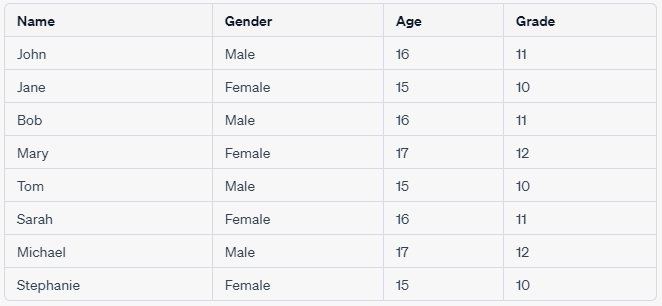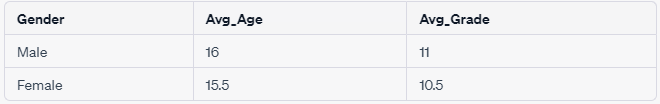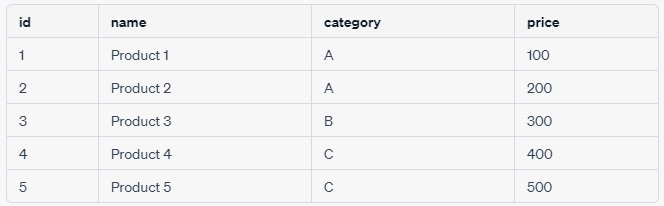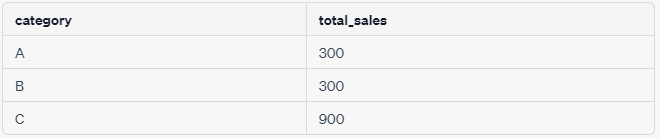Total Page Preview:   000000000317

## SQL group by function in Engilsh

The SQL GROUP BY function is used to group the rows of a result set based on one or more columns. This is often used in conjunction with an aggregate function like SUM, COUNT, AVG, etc., to calculate summary statistics for each group.

here's an example of how the SQL GROUP BY function works using a tabular example:
Suppose we have a table called students that contains information about the students in a school, including their names, genders, ages, and grades. The table might look something like this:Now, suppose we want to find out the average age and grade of the students by gender. We can use the GROUP BY function to group the rows by gender and then calculate the average age and grade for each group. The SQL query for this would look like:The result set would look like this:In this example, we've grouped the rows by Gender, and then used the AVG function to calculate the average Age and Grade for each group. The result set shows that the average age of male students is 16, while the average age of female students is 15.5. Similarly, the average grade of male students is 11, while the average grade of female students is 10.5.

## SQL group by function in Hindi

SQL GROUP BY function ka upyog ek table ke rows ko ek ya adhik columns ke aadhar par group karne ke liye kiya jaata hai. Iska istemal generally aggregate function jaise SUM, COUNT, AVG ke saath kiya jaata hai, jisse har group ke liye summary statistics calculate kiye jaate hain. Yahaan ek tabular example ke saath iska istemal samajhaya gaya hai:
Suppose humare paas ek table hai sales jismein yeh columns hain:
• id (integer)
• name (text)
• category (text)
• price (integer)
Table ka data is tarah hai:Hum chahte hain ki har category ke liye total sales amount calculate ho. Iske liye, hum is query ka istemal kar sakte hain:Iss query mein GROUP BY clause category column ke aadhar par table ke rows ko group karta hai aur SUM function ka upyog har category ke total sales amount calculate karne ke liye kiya jaata hai. AS keyword ka upyog calculated column ke naam dene ke liye kiya jaata hai, jiska naam is example mein total_sales hai.

Result set is tarah hoga:Iss result set se hum dekh sakte hain ki A category ke total sales amount 300 hai, B category ke total sales amount bhi 300 hai aur C category ke total sales amount 900 hai.

Thank You#### Brijesh Kumar

Database Developer

I have more then 6 years Experience in Microsoft Technologies - SQL Server Database, ETL Azure Cloud - Azure SQL Database, CosmosDB, Azure Data Factory, PowerBI, Web Job, Azure Function, Azure Storage, Web Apps, Powershall and Database Migration On-Premise to Azure Cloud.